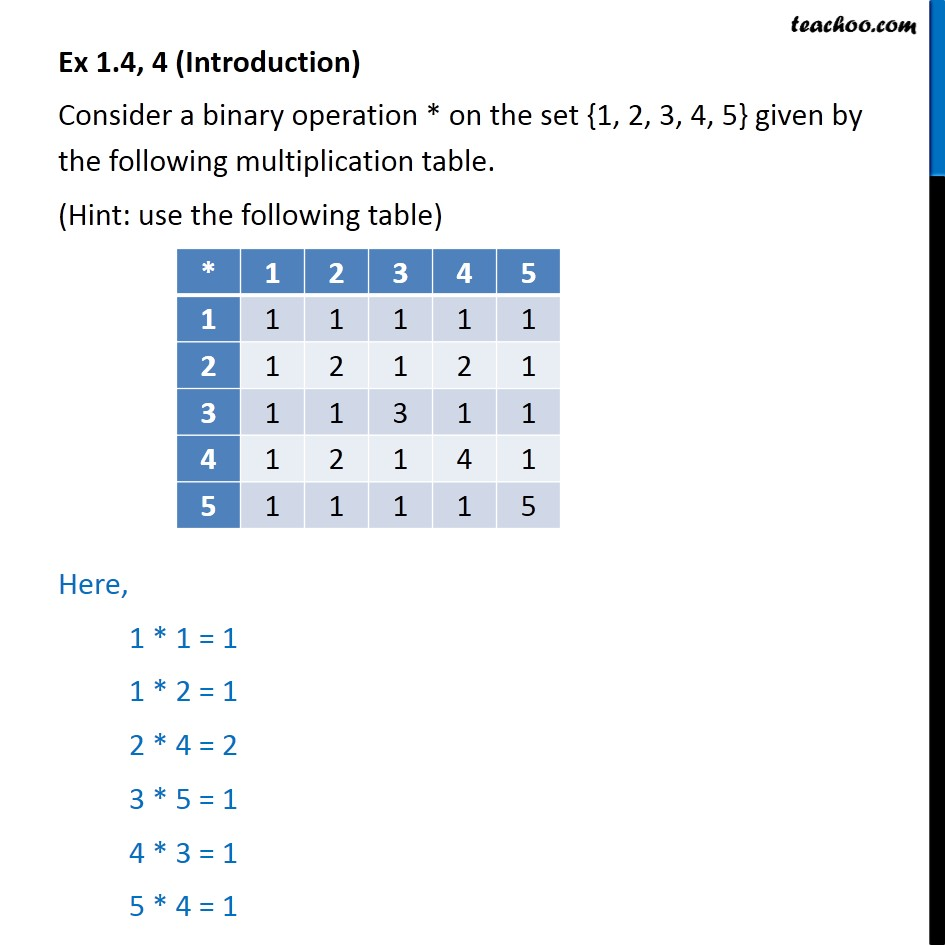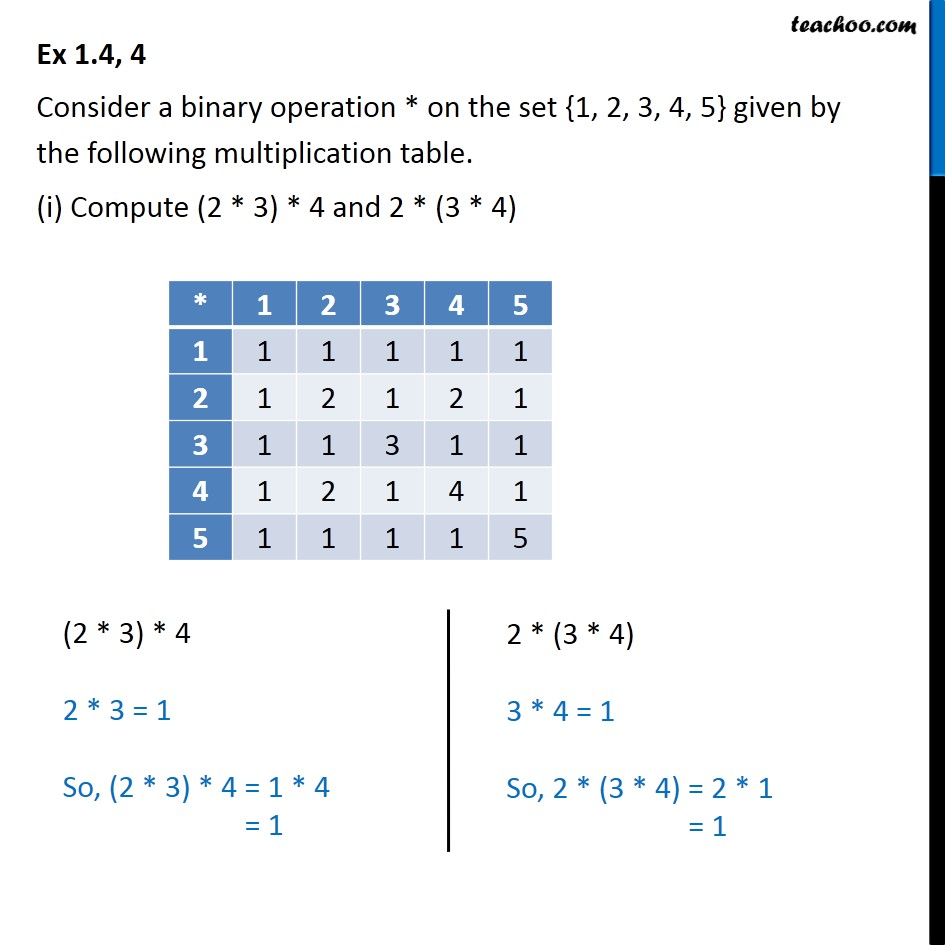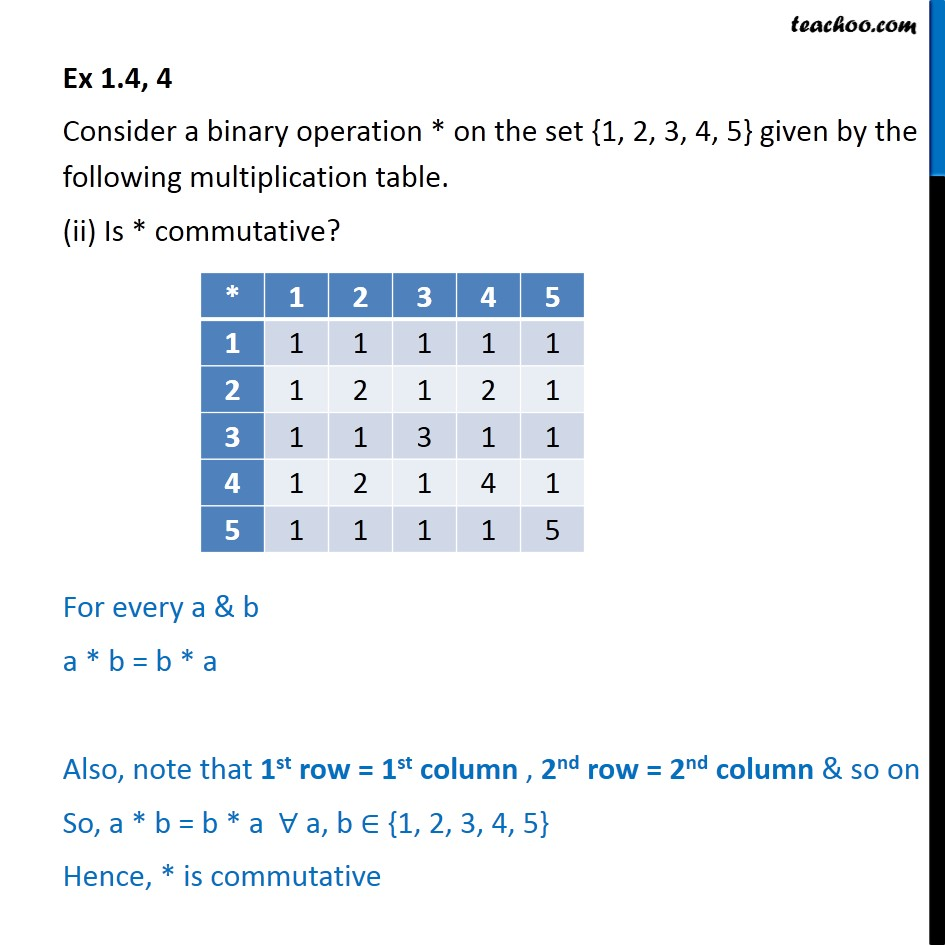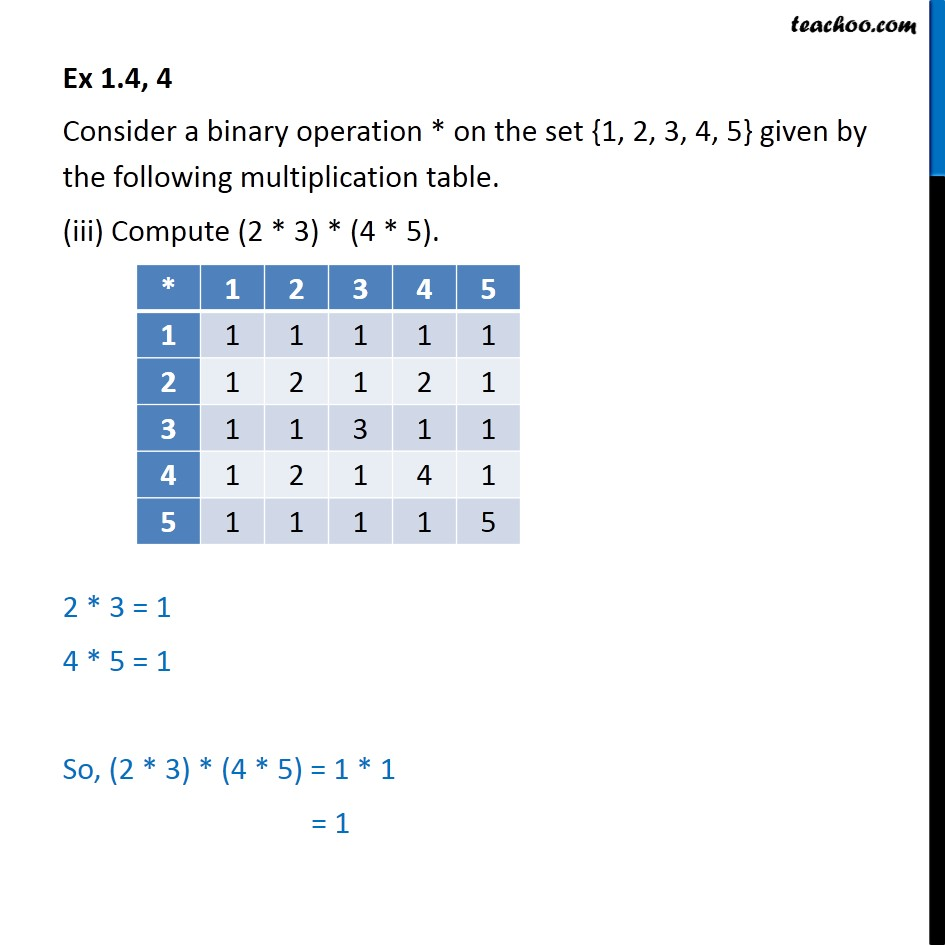Whether binary commutative/associative or not

Chapter 1 Class 12 Relation and Functions
Concept wiseLearn in your speed, with individual attention - Teachoo Maths 1-on-1 Class

### Transcript

Ex 1.4, 4 (Introduction) Consider a binary operation * on the set {1, 2, 3, 4, 5} given by the following multiplication table. (Hint: use the following table) Here, 1 * 1 = 1 1 * 2 = 1 2 * 4 = 2 3 * 5 = 1 4 * 3 = 1 5 * 4 = 1 Ex 1.4, 4 Consider a binary operation * on the set {1, 2, 3, 4, 5} given by the following multiplication table. (i) Compute (2 * 3) * 4 and 2 * (3 * 4) Ex 1.4, 4 Consider a binary operation * on the set {1, 2, 3, 4, 5} given by the following multiplication table. (ii) Is * commutative? For every a & b a * b = b * a Also, note that 1st row = 1st column , 2nd row = 2nd column & so on So, a * b = b * a a, b {1, 2, 3, 4, 5} Hence, * is commutative Ex 1.4, 4 Consider a binary operation * on the set {1, 2, 3, 4, 5} given by the following multiplication table. (iii) Compute (2 * 3) * (4 * 5). 2 * 3 = 1 4 * 5 = 1 So, (2 * 3) * (4 * 5) = 1 * 1 = 1